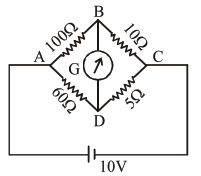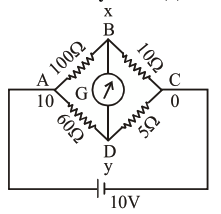# The four arms of a Wheatstone`
Question:

The four arms of a Wheatstone bridge have resistances as shown in the figure. A galvanometer of $15 \Omega$ resistance is connected across BD. Calculate the current through the galvanometer when a potential difference of $10 \mathrm{~V}$ is maintained across AC.1. $2.44 \mu \mathrm{A}$

2. $2.44 \mathrm{~mA}$

3. $4.87 \mathrm{~mA}$

4. $4.87 \mu \mathrm{A}$

Correct Option: , 3

Solution:$\frac{x-10}{100}+\frac{x-y}{15}+\frac{x-0}{10}=0$

$53 x-20 y=30$..(1)

$\frac{y-10}{60}+\frac{y-x}{15}+\frac{y-0}{5}=0$

$17 y-4 x=10$..(2)

on solving (1) \& (2)

$x=0.865$

$y=0.792$

$\Delta \mathrm{V}=0.073 \mathrm{R}=15 \Omega$

$\mathrm{i}=4.87 \mathrm{~mA}$